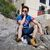# Standardising rasters in arcpy

298
3
Jump to solution
05-14-2021 06:15 AMNew Contributor III

Hello

I have to to standardise some rasters using a loop and following equation

X1= (X - X.min())/(X.max()-X.min())

where X is the original dataset, and X1 the new standardized dataset, which has a minimum value of 0 and a maximum value of 1.

I was wondering how I would go about doing this? the current code I have is

``````for calc in projected_rast:
print calc
minLSTresult = arcpy.GetRasterProperties_management(calc, "MINIMUM")
maxLSTresult = arcpy.GetRasterProperties_management(calc, "MAXIMUM")
standardised = ((calc - minLSTresult)/(maxLSTresult-minLSTresult))
standardised.save()``````

were projected_rast is a list of strings to my rasters

Tags (3)
1 Solution

Accepted SolutionsbyMVP Regular Contributor

a Raster object has maximum and minimum properties (if statistics have been calculated).

``````for calc in projected_rast:
print(calc)
raster = arcpy.Raster(calc)
minLSTresult = raster.minimum
maxLSTresult = raster.maximum
standardised = ((raster - minLSTresult)/(maxLSTresult-minLSTresult))
standardised.save()``````
3 RepliesbyMVP Honored Contributor

What does it yield?

Are you using ArcMap? (your print statement suggests python 2.7)

Do you have any null areas/values?

Of course you could use RasterToNumPyArray and use numpy to do the work quickly.

``````import numpy as np
a = np.arange(0, 12.).reshape(4, 3)
a
array([[ 0.,  1.,  2.],
[ 3.,  4.,  5.],
[ 6.,  7.,  8.],
[ 9., 10., 11.]])

a_min = np.nanmin(a)
a_max = np.nanmax(a)
a_stand = (a - a_min) / (a_max - a_min)

a_stand
array([[0.        , 0.09090909, 0.18181818],
[0.27272727, 0.36363636, 0.45454545],
[0.54545455, 0.63636364, 0.72727273],
[0.81818182, 0.90909091, 1.        ]])``````

... sort of retired...byMVP Regular Contributor

a Raster object has maximum and minimum properties (if statistics have been calculated).

``````for calc in projected_rast:
print(calc)
raster = arcpy.Raster(calc)
minLSTresult = raster.minimum
maxLSTresult = raster.maximum
standardised = ((raster - minLSTresult)/(maxLSTresult-minLSTresult))
standardised.save()``````New Contributor III

Worked perfectly 🙂